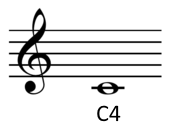# Convert pitch between Hz, Barks, and music notation

Fill in one value and leave the other two blank. Click the Convert button, and the missing values will be filled in.

 Cycles per second (Hz) Critical band (Bark scale) Music notation (e.g. A5, F#2, Eb4, etc.)

If more than one box has a valid entry, the first one is used.

## Details

### Hertz

The frequency of a pitch in Hertz (Hz) is the number of cycles per second. For example, an A 440 has a pitch of 440 Hz, 440 vibrations per second.

### Bark scale

The Bark scale is a psychoacoustic measure of frequency, dividing the audible range into 24 bands that are equal in a physiological sense having to do with the human hearing system. The unit "Bark" was named in honor of acoustician Heinrich Barkhausen.

There are several slightly different ways to convert between frequency in Hz and Barks. This page uses

z = 6 arcsinh(f/600)

to convert frequency f in Hz to critical band rate z in Barks.

The inverse formula is

f = 600 sinh(z/6).

### Musical notation

This page uses so-called scientific pitch notation. The C near the lower threshold of hearing is denoted C0. The C one octave higher is C1. The C two octaves above C0 is C2, etc. Middle C is C4.Notes besides C's take their octave number from the nearest C below. For example, the lowest note on a standard piano is A0, the A above C0. More details here.

In the form above use 'b' (the second letter of the English alphabet) for flat symbol ♭. So, for example, Eb4 is the E-flat above middle C.

If a frequency doesn't correspond exactly to a chromatic note, the difference is given in cents, where a cent is a hundredth of a semitone. For example, 440 Hz is A4 and 450 Hz is A4 + 39 cents.

More calculators like this one.Is there anything else I can help you with? Maybe something with acoustics?